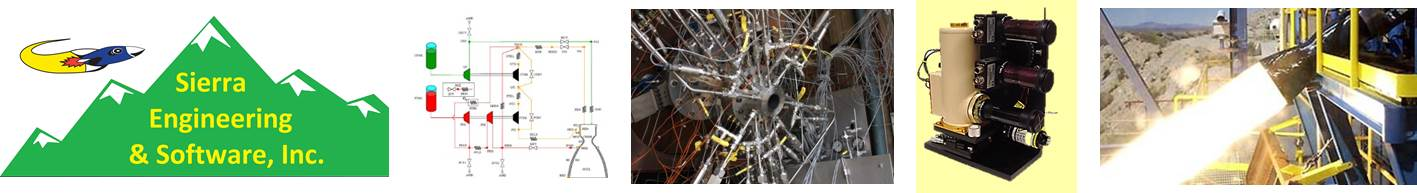## Chemical Equilibrium and Applications (CEA) Program

#### Description

Calculates the theoretical thermodynamic properties for an equilibrium mixture. The program can be applied to equipment such as compressors, turbines, nozzles, engines, shock tubes, heat exchangers, and chemical processing equipment.

#### Pricing

Year - \$500
Month - \$30
Week - \$20
Day - \$15
Initiation Fee - \$200
Export controlledThe program can handle any of the following, specific problems: (a) Calculate chemical equilibrium composition for thermodynamic states (b) Calculate theoretical rocket performance for finite or infinite area combustion chamber (c) Calculate Champman-Jouguet detonations (d) Calculate shock tube parameters for both incident and reflected shocks To calculate the equilibrium composition of a composition, the user is required to supply the state of the equilibrium mixture. The user provides the program with any two thermodynamic-state functions from the following list: Temperature and pressure Enthalpy and pressure Entropy and pressure Temperature and volume (or density) Internal energy and volume (or density) Entropy and volume (or density) The shock and detonation problems require the use of input data of types (1) and (2). Solving the rocket problem requires the input of data type (3) and type (1) or (2). The thermodynamic data calculated by the program include conductivity, viscosity, specific heats, sound speed, equilibrium temperature and pressure, enthalpy, entropy, internal energy, and many others.